# Math Planes Menu Help

#### Fractal Zoomer

Math Planes Menu
•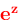, transforms the initial complex number, by using the transformation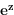.
•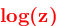, transforms the initial complex number, by using the transformation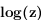.
•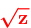, transforms the initial complex number, by using the transformation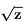.
•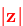, transforms the initial complex number, by using the transformation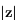(absolute value).
•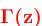, transforms the initial complex number, by using the gamma function tranformation.
•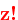, transforms the initial complex number, by using the factorial transformation.
•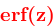, transforms the initial complex number, by using the error function transformation.
•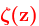, transforms the initial complex number, by using the riemann zeta function transformation.

• Trigonometric Planes Menu, containts all the trigonometric transformations.

• Inverse Trigonometric Planes Menu, contains all the inverse trigonometric transformations.